Vocabulary Quick Reference MathBitsNotebook.com Terms of Use   Contact Person: Donna RobertsThis is a partial listing of basic FORMAL definitions associated with segments, angles, and triangles associated with geometry. You need to have a thorough understanding of these terms.
(This is an alphabetic listing.)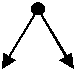acute angle An acute angle is an angle whose measure is greater than 0° and less than 90º.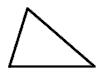acute triangle An acute triangle is a triangle that has three acute angles.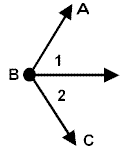∠1 and ∠2 are adjacent ∠1 and ∠ABC are not adjacent Adjacent angles share a common vertex, a common side and no common interior points (they do not overlap).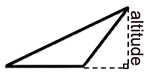altitude for an obtuse triangle The altitude (height) of a triangle is a line segment extending from any vertex of a triangle perpendicular to the line containing the opposite side.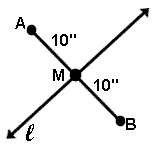M is the midpoint of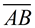Bisector of a line segment is any line (or subset of a line) that intersects the segment at its midpoint. Alternate definition:  Bisector of a line segment is any line (or subset of a line) that divides the segment into two congruent segments.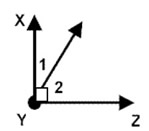∠1 and ∠2 are complementary Complementary angles are two angles the sum of whose measures is 90º.  (Complementary angles need not be adjacent angles.)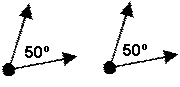congruent angles Congruent angles are angles of the same measure.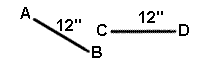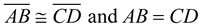Congruent segments are segments of the same length (measure).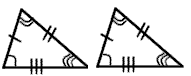congruent triangles Congruent triangles are triangles whose corresponding angles and sides are congruent.  (They are exactly the same size and shape.)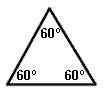equiangular triangle An equiangular triangle is a triangle which has three congruent angles.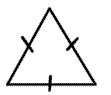equilateral triangle An equilateral triangle is a triangle with three congruent sides.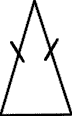isosceles triangle An isosceles triangle is a triangle with two congruent sides.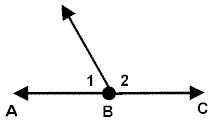linear pair A linear pair of angles are adjacent angles whose non-common sides are opposite rays (form a straight line).  The sum of the measures of the angles in a linear pair is 180º.  Unlike supplementary angles, a linear pair MUST be two adjacent angles.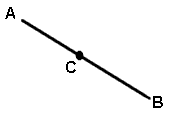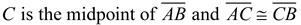Midpoint of a line segment is the point on that line segment that divides the segment two congruent segments.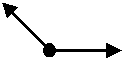obtuse angle An obtuse angle is an angle whose measure is greater than 90º and less than 180º.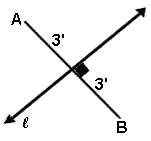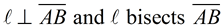The perpendicular bisector of a segment is a line (or subset of a line) that bisects the segment and is perpendicular to the segment.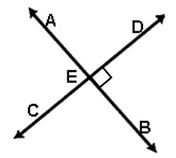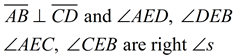Perpendicular lines are two lines which intersect to form right angles.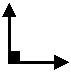right angle A right angle is an angle whose measure is 90º.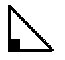right triangle A right triangle is a triangle containing one right angle.scalene triangle A scalene triangle is a triangle with no congruent sides.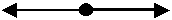straight angle A straight angle is an angle whose measure is 180º. It looks like a straight line.∠1 and ∠2 are supplementary Supplementary angles are two angles the sum of whose measures is 180º.  (Supplementary angles need not be adjacent.)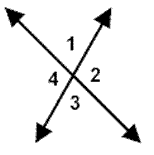∠1 and ∠3 are vertical angles ∠2 and ∠4 are vertical angles Vertical angles are two nonadjacent angles formed by two intersecting lines. Vertical angles are equal in measure.